Chapter 5, Problem 5.28QP### General Chemistry - Standalone boo...

11th Edition
Steven D. Gammon + 7 others
ISBN: 9781305580343

#### Solutions

Chapter
Section### General Chemistry - Standalone boo...

11th Edition
Steven D. Gammon + 7 others
ISBN: 9781305580343
Textbook Problem

# Gas Laws and Kinetic Theory of Gases IIConsider the box below that contains a single atom of an ideal gas. a Assuming that this gas atom is moving, describe how it creates pressure inside the container.b Now consider the two containers below, each at the same temperature. If we were to measure the gas pressure in each container, how would they compare? Explain your answer.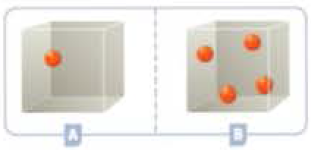c Consider the two containers below, labeled C and D, one with an ideal gas atom and one with ideal gas molecules, each at the same temperature. The gas molecule has more mass than the gas atom. How do the pressures of the two containers compare? Why?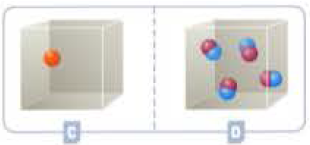d For the containers C and D above, which would have the higher root-mean-square (rms) molecular speed? How does the difference in rms speeds support or contradict the answer you obtained above when you compared the pressures? e Consider containers E and F below. How do the pressures in these containers compare? Be sure to explain your answer.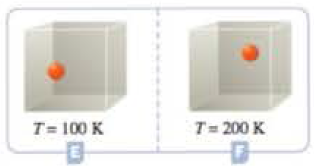f Consider containers G and H below, one with a gas atom and one with a gas molecule. As before, the gas molecule has more mass than the gas atom. Explain how the pressures in these containers compare.g Now think about the containers below, I and J. How do the pressures in these containers compare? Be sure to justify your answer.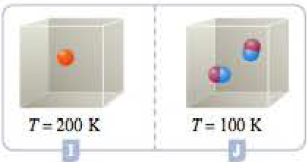h Finally, how do the pressures in containers K and L compare?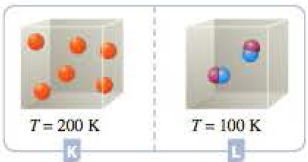(a)

Interpretation Introduction

Interpretation:

The amount of pressure created when a gas molecule moving in a closed container has to be explained.

Concept Introduction:

Gas pressure

Pressure or Stress is the force applied perpendicular to the surface of an object per unit area.

SI derived unit of pressure is Pascal (Pa).

Explanation

Figure 1

According to kinetic theory of gases the molecules in a container are continuously moving freely...

(b)

Interpretation Introduction

Interpretation:

The amount of pressure created when the gas molecule(s) moving in closed containers A and B has to be explained.

Concept Introduction:

Gas pressure:

Pressure or Stress is the force applied perpendicular to the surface of an object per unit area.

SI derived unit of pressure is Pascal (Pa).

(c)

Interpretation Introduction

Interpretation:

The amount of pressure has to be explained when the containers C and D contains atoms and molecules.

Concept Introduction:

Gas pressure:

Pressure or Stress is the force applied perpendicular to the surface of an object per unit area.

SI derived unit of pressure is Pascal (Pa).

(d)

Interpretation Introduction

Interpretation:

Among the given containers C and D, the container with highest RMS value has to be identified and answer has to be contradicted with the pressure of the container.

Concept Introduction:

Root mean square value:

μrms=3RTMm

Where,

μrms=rootmeansquarevalueR= idealgasconstant = 8.314joulemole.KelvinK=absolutetemperatureinkelvinM=molarmassofthemoleculeinkilograms

(e)

Interpretation Introduction

Interpretation:

At the given conditions the pressures among the Containers E and F should be compared.

Concept Introduction:

Gay-Lussac’s law:

Gay-Lussac law derived from the Charles law,

The law states that a sample gas having fixed pressure is directly proportional to the temperature. Since pressure can never be negative and the temperature scale should be absolute minimum.

PαTPT = constant

So the initial and final Pressure will be like

P2T2 = P1T1

Here,

P1 and P2 are initial and final Pressure.

T1 and T2 are initial and final Temperature.

(f)

Interpretation Introduction

Interpretation:

At the given conditions the pressures among the Containers G and H should be compared.

Concept Introduction:

Gay-Lussac’s law:

Gay-Lussac law derived from the Charles law,

The law states that a sample gas having fixed pressure is directly proportional to the temperature. Since pressure can never be negative and the temperature scale should be absolute minimum.

PαTPT = constant

So the initial and final Pressure will be like

P2T2 = P1T1

Here,

P1 and P2 are initial and final Pressure.

T1 and T2 are initial and final Temperature.

(g)

Interpretation Introduction

Interpretation:

At the given conditions the pressures among the Containers G and H should be compared.

Concept Introduction:

Gay-Lussac’s law:

Gay-Lussac law derived from the Charles law,

The law states that a sample gas having fixed pressure is directly proportional to the temperature. Since pressure can never be negative and the temperature scale should be absolute minimum.

PαTPT = constant

So the initial and final Pressure will be like

P2T2 = P1T1

Here,

P1 and P2 are initial and final Pressure.

T1 and T2 are initial and final Temperature.

(h)

Interpretation Introduction

Interpretation:

At the given conditions the pressures among the Containers K and L should be compared.

Concept Introduction:

Gay-Lussac’s law:

Gay-Lussac law derived from the Charles law,

The law states that a sample gas having fixed pressure is directly proportional to the temperature. Since pressure can never be negative and the temperature scale should be absolute minimum.

PαTPT = constant

So the initial and final Pressure will be like

P2T2 = P1T1

Here,

P1 and P2 are initial and final Pressure.

T1 and T2 are initial and final Temperature.

### Still sussing out bartleby?

Check out a sample textbook solution.

See a sample solution

#### The Solution to Your Study Problems

Bartleby provides explanations to thousands of textbook problems written by our experts, many with advanced degrees!

Get Started

## Additional Science Solutions

#### Find more solutions based on key concepts